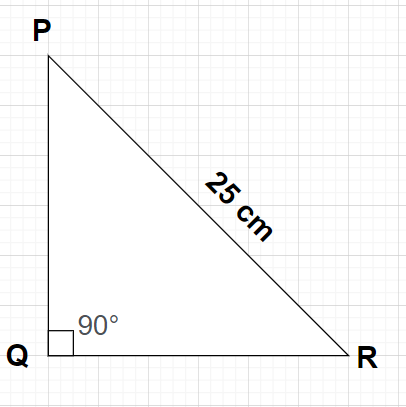QUESTION

# The perimeter of a right triangle is 60 cm, its hypotenuse is 25 cm. Find the area of the triangle.

Hint: Here, we will proceed by firstly finding all the sides of the triangle PQR using the concept that the perimeter of any triangle is equal to the sum of all the sides and then we will use the formula for the area of any triangle i.e., Area of the triangle = $\dfrac{1}{2} \times$(Base)$\times$(Height).Let us suppose a right triangle PQR which is right angled at vertex Q.
Given, Perimeter of the right triangle PQR = 60 cm
Hypotenuse of the right triangle PQR, PR = 25 cm
Since, the perimeter of any triangle is equal to the sum of all the three sides of the triangle.
i.e., Perimeter of the right triangle PQR = PQ + QR + PR
$\Rightarrow$60 = PQ + QR + 25
$\Rightarrow$PQ +QR = 60 -25
$\Rightarrow$PQ +QR = 35
$\Rightarrow$PQ = (35 – QR) $\to (1)$
By Pythagoras Theorem in any right triangle, we have
${\left( {{\text{Hypotenuse}}} \right)^2} = {\left( {{\text{Perpendicular}}} \right)^2} + {\left( {{\text{Base}}} \right)^2}$
Using the Pythagoras Theorem in right triangle PQR, we get
${\left( {{\text{PR}}} \right)^2} = {\left( {{\text{PQ}}} \right)^2} + {\left( {{\text{QR}}} \right)^2}$
By putting PR = 25 and substituting equation (1) in the above equation, we get
$\Rightarrow {\left( {{\text{25}}} \right)^2} = {\left( {{\text{35}} - {\text{QR}}} \right)^2} + {\left( {{\text{QR}}} \right)^2} \\ \Rightarrow 625 = {\left( {{\text{35}}} \right)^2} + {\left( {{\text{QR}}} \right)^2} - 2\left( {35} \right)\left( {{\text{QR}}} \right) + {\left( {{\text{QR}}} \right)^2} \\ \Rightarrow 2{\left( {{\text{QR}}} \right)^2} - 70\left( {{\text{QR}}} \right) - 625 + 1225 = 0 \\ \Rightarrow 2{\left( {{\text{QR}}} \right)^2} - 70\left( {{\text{QR}}} \right) + 600 = 0 \\ \Rightarrow {\left( {{\text{QR}}} \right)^2} - 35\left( {{\text{QR}}} \right) + 300 = 0 \\ \Rightarrow {\left( {{\text{QR}}} \right)^2} - 15\left( {{\text{QR}}} \right) - 20\left( {{\text{QR}}} \right) + 300 = 0 \\ \Rightarrow \left( {{\text{QR}}} \right)\left( {{\text{QR}} - 15} \right) - 20\left( {{\text{QR}} - 15} \right) = 0 \\ \Rightarrow \left( {{\text{QR}} - 15} \right)\left( {{\text{QR}} - 20} \right) = 0 \\$
Either ${\text{QR}} - 15 = 0 \\ \Rightarrow {\text{QR}} = 15{\text{ cm}} \\$ or ${\text{QR}} - 20 = 0 \\ \Rightarrow {\text{QR}} = 20{\text{ cm}} \\$
Put QR = 15 cm in equation (1), we get
$\Rightarrow$PQ = (35 – 15) = 20 cm
Put QR = 20 cm in equation (1), we get
$\Rightarrow$PQ = (35 – 20) = 15 cm
As, we know that the area of any triangle is given by
Area of the triangle = $\dfrac{1}{2} \times$(Base)$\times$(Height)
For right angled triangle PQR with PQ = 20 cm, QR = 15 cm and PR = 25 cm,
Base = QR = 15 cm, Height = PQ = 20 cm
So, Area of the triangle PQR = $\dfrac{1}{2} \times$(QR)$\times$(PQ) = $\dfrac{1}{2} \times$(15)$\times$(20) = 150 ${\text{c}}{{\text{m}}^2}$
For right angled triangle PQR with PQ = 15 cm, QR = 20 cm and PR = 25 cm,
Base = QR = 15 cm, Height = PQ = 20 cm
So, Area of the triangle PQR = $\dfrac{1}{2} \times$(QR)$\times$(PQ) = $\dfrac{1}{2} \times$(20)$\times$(15) = 150 ${\text{c}}{{\text{m}}^2}$
In both the cases, the value of the area of the triangle PQR obtained is the same which is equal to 150 ${\text{c}}{{\text{m}}^2}$.

Note: In any right angled triangle, the side opposite to the right angle is known as the hypotenuse, the side opposite to the considered acute angle is known as the perpendicular and the remaining side is known as the base. Here, in this case the hypotenuse is the side PR, the perpendicular and the base of this triangle will depend on the considered acute angle.